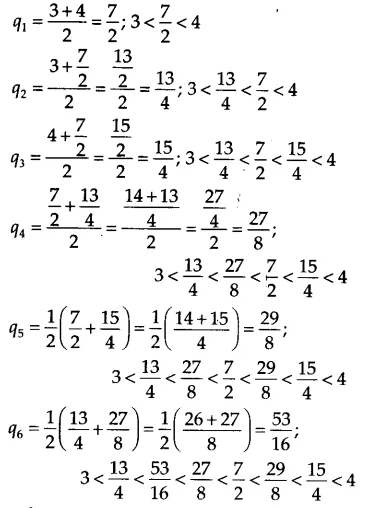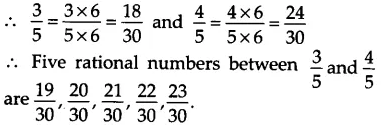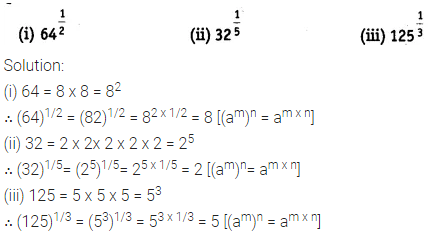# NCERT Solutions for Class 9 Maths Chapter 1 Number Systems

NCERT Solutions for Class 9 Maths Chapter 1 Number Systems Ex 1.1 are part of NCERT Solutions for Class 9 Maths . Here we have given NCERT Solutions for Class 9 Maths Chapter 1 Number Systems Ex 1.1.

## NCERT Solutions for Class 9 Maths Chapter 1 Number Systems Ex 1.1

### Ex 1.1 Class 9 Maths Question 1.

Is zero a rational number? Can you write it in the form p/q,where p and q are integers and q ≠0?

Solution:

Yes, zero is a rational number it can be written in the form p/q

0=0/1=0/2=0/3 etc. denominator q can also be taken as negative integer.

### Ex 1.1 Class 9 Maths Question 2.

Find six rational numbers between 3 and 4.

Solution:

Let qi be the rational number between 3

and 4, where j = 1 to 6.

∴ Six rational numbers are as follows:Thus, the six rational numbers between 3 and 4 are

7/2,13/4,15/4,27/8,29/8 and 53/16.

### Ex 1.1 Class 9 Maths Question 3.

Find five rational numbers between3/5 and 4/5

Solution:

Since, we need to find five rational numbers, therefore, multiply numerator and denominator by 6.### Ex 1.1 Class 9 Maths Question 4.

State whether the following statements are true or false. Give reasons for your answers.

(i) Every natural number is a whole number.

(ii) Every integer is a whole number.

(iii) Every rational number is a whole number.

Solution:

(i) True

∴ The collection of all natural numbers and 0 is called whole numbers.

(ii) False

∴ Negative integers are not whole numbers.

(iii) False

∴ Rational numbers are of the form p/q, q ? 0 and q does not divide p completely that are not whole numbers.

## NCERT Solutions for Class 9 Maths Chapter 1 Number Systems Ex 1.2

### Ex 1.2 Class 9 Maths Question 1.

State whether the following statements are true or false. Justify your answers.

(i) Every irrational number is a real number.

(ii) Every point on the number line is of the form vm , where m is a natural number.

(iii) Every real number is an irrational number.

Solution:

(i) True

Because all rational numbers and all irrational numbers form the group (collection) of real numbers.

(ii) False

Because negative numbers cannot be the square root of any natural number.

(iii) False

Because rational numbers are also a part of real numbers.

### Ex 1.2 Class 9 Maths Question 2.

Are the square roots of all positive integers irrational? If not, give an example of the square root of a number that is a rational number.

Solution:

No, if we take a positive integer, say 9, its square root is 3, which is a rational number.

### Ex 1.2 Class 9 Maths Question 3.

Show how √5 can be represented on the number line.

Solution:

Draw a number line and take point O and A on it such that OA = 1 unit. Draw BA ⊥ OA as BA = 1 unit. Join OB = √5 units.## NCERT Solutions for Class 9 Maths Chapter 1 Number Systems Ex 1.3

### Ex 1.3 Class 9 Maths Question 1.

Write the following in decimal form and say what kind of decimal expansion each has### Ex 1.3 Class 9 Maths Question 2.## NCERT Solutions for Class 9 Maths Chapter 1 Number Systems Ex 1.4

### Ex 1.4 Class 9 Maths Question 1.

Visualise 3.765 on the number line, using successive magnification.

Solution:

3.765 lies between 3 and 4.### Ex 1.4 Class 9 Maths Question 2.## NCERT Solutions for Class 9 Maths Chapter 1 Number Systems Ex 1.5

### Ex 1.5 Class 9 Maths Question 1.

Classify the following numbers as rational or irrational.### Ex 1.5 Class 9 Maths Question 2.

Simplify each of the following expressions### Ex 1.5 Class 9 Maths Question 3.## NCERT Solutions for Class 9 Maths Chapter 1 Number Systems Ex 1.6

### Ex 1.6 Class 9 Maths Question 1.

Find### Ex 1.6 Class 9 Maths Question 2.

Find### Ex 1.6 Class 9 Maths Question 3.

Simplify:Your mobile number will not be published.• Mayank
• 2021-March-26 11:24:00
Ok• Ramu
• 2020-May-05 15:49:15
NCERT Solutions For Class 11